# Chapter 4 Graphing Linear Equations and Functions Algebra

• Slides: 9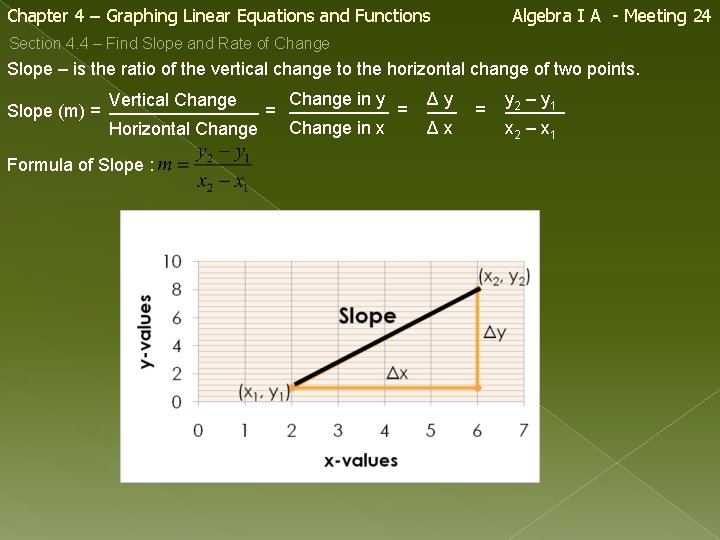Chapter 4 – Graphing Linear Equations and Functions Algebra I A - Meeting 24 Section 4. 4 – Find Slope and Rate of Change Slope – is the ratio of the vertical change to the horizontal change of two points. Change in y Vertical Change Slope (m) = ________ = Change in x Horizontal Change Formula of Slope : Δ y ___ = Δx y______ 2 – y 1 x 2 – x 1Chapter 4 – Graphing Linear Equations and Functions Section 4. 4 – Find Slope and Rate of Change Example # 1 Find the slope of the line shown. Let (x 1, y 1) = (-2, -2) and (x 2, y 2) = (6, 4) Write formula for Slope : Substitute: Simplify: The slope of the line is Algebra I A - Meeting 24Chapter 4 – Graphing Linear Equations and Functions Section 4. 4 – Find Slope and Rate of Change Example # 2 Find the slope of the line shown. Let (x 1, y 1) = (-2, 2) and (x 2, y 2) = (3, 1) Write formula for Slope : Substitute: Simplify: The slope of the line is Algebra I A - Meeting 24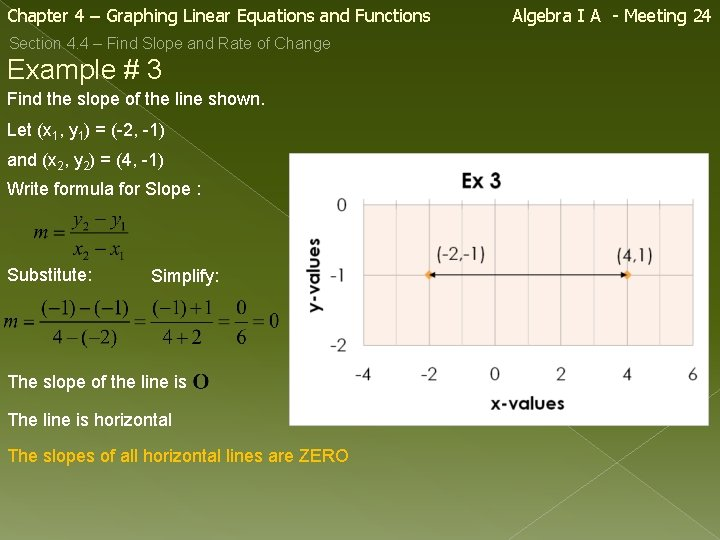Chapter 4 – Graphing Linear Equations and Functions Section 4. 4 – Find Slope and Rate of Change Example # 3 Find the slope of the line shown. Let (x 1, y 1) = (-2, -1) and (x 2, y 2) = (4, -1) Write formula for Slope : Substitute: Simplify: The slope of the line is The line is horizontal The slopes of all horizontal lines are ZERO Algebra I A - Meeting 24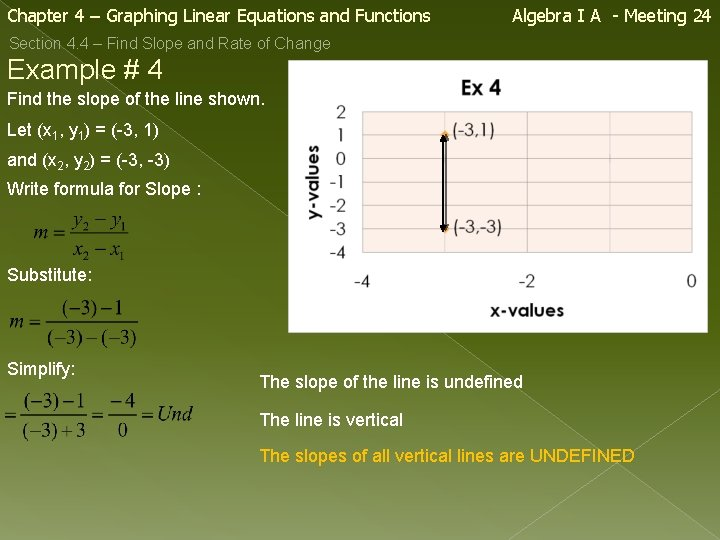Chapter 4 – Graphing Linear Equations and Functions Algebra I A - Meeting 24 Section 4. 4 – Find Slope and Rate of Change Example # 4 Find the slope of the line shown. Let (x 1, y 1) = (-3, 1) and (x 2, y 2) = (-3, -3) Write formula for Slope : Substitute: Simplify: The slope of the line is undefined The line is vertical The slopes of all vertical lines are UNDEFINEDChapter 4 – Graphing Linear Equations and Functions Algebra I A - Meeting 24 Section 4. 4 – Find Slope and Rate of Change – compares a change in one quantity to a change in another quantity. Example # 5 The table shows the cost to paint a house for a given number of hours. Find the rate of change in cost with respect to time. Time (hours) 4 6 8 Cost (\$) 90 135 180 Change in Cost Rate of Change = _______ Change in Time Trial 1 : = \$22. 50 per hour Trial 2 : = \$22. 50 per hour Trial 3 : = \$22. 50 per hour The trials confirm, the Rate of Change is constant. The rate of change is \$22. 50 per hour.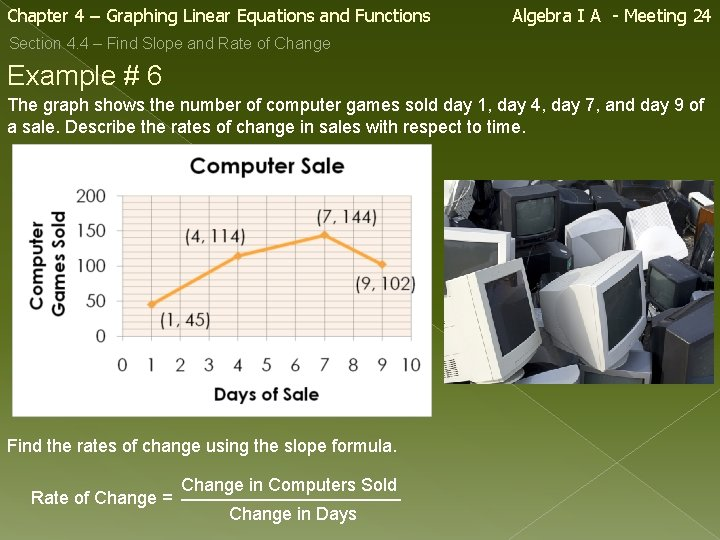Chapter 4 – Graphing Linear Equations and Functions Algebra I A - Meeting 24 Section 4. 4 – Find Slope and Rate of Change Example # 6 The graph shows the number of computer games sold day 1, day 4, day 7, and day 9 of a sale. Describe the rates of change in sales with respect to time. Find the rates of change using the slope formula. Change in Computers Sold Rate of Change = ___________ Change in DaysChapter 4 – Graphing Linear Equations and Functions Algebra I A - Meeting 24 Section 4. 4 – Find Slope and Rate of Change Example # 6 (con’t) Describe the rates of change in sales with respect to time. Days 1 - 4 : Sales increased at a fast rate. Days 4 - 7 : Sales increased at a slower rate. Days 7 - 9 : Sales decreased at a moderate. Rate of Change Days 1 - 4 : = 23 computers sold per day. Days 4 - 7 : = 10 computers sold per day. Days 7 - 9 : = -21 computers sold per day.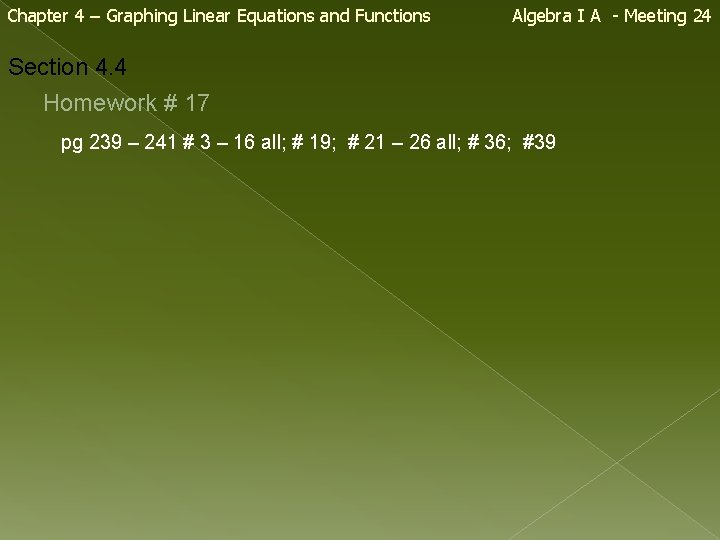Chapter 4 – Graphing Linear Equations and Functions Algebra I A - Meeting 24 Section 4. 4 Homework # 17 pg 239 – 241 # 3 – 16 all; # 19; # 21 – 26 all; # 36; #39